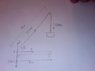# Solve Crane Statics Problem: Draw FBD & Find Min Angle

• Wilko81
Thanks for the help!In summary, the problem states that a crane of mass 30,000kg is secured to the end of a steel plate 6 m long. At each end of the plate are a set of wheels. The crane has a jib of length 15m and is required to lift a mass of 25,000kgs from the end of the jib. The jib is positioned over the 6m long steel plate. The tension in the cable is the same as the weight of the lifted mass, m*g, where g = 9.81 m/s^2. Your value is incorrect. Try again.

#### Wilko81

Hi, the problem states;

A crane of mass 30,000kg is secured to the end of a steel plate 6 m long. At each end of the plate are a set of wheels. The crane has a jib of length 15m and is required to lift a mass of 25,000kgs from the end of the jib. The jib is positioned over the 6m long steel plate.

Draw a free body diagram.
What is the minimum angle with the horizontal that the jib can be, without the crane falling over?

I've attempted the free body diagram (the forces need scaling up by 10), I'm pretty sure it's not correct, any pointers in the right direction would be appreciated.

Thanks

#### Attachments

•Photo 15.jpg
12 KB · Views: 882
Remember wheels have a force exerted on them... and the cable should be tensioned against the load.

Cheers,
Colt 45 J

Is the load distributed evenly over the 2 sets of wheels i.e. 150kN over each?

I've indicted on the attached picture 250kN for the tension in the cable. Is this correct?

Welcome to PF!

Hi Wilko81! Welcome to PF!Wilko81 said:
Is the load distributed evenly over the 2 sets of wheels i.e. 150kN over each?

Certainly not.

And when the crane is about to tip over, the load on the real wheel will be zero, won't it?I've indicted on the attached picture 250kN for the tension in the cable. Is this correct?

The tension is an internal force, and has nothing to do with this problem.

You need only consider external forces.Wilko81 said:
I've attempted the free body diagram (the forces need scaling up by 10), I'm pretty sure it's not correct, any pointers in the right direction would be appreciated.

erm… that isn't a free body diagram …

an fbd is a diagram with all the external forces marked on it (with arrows) at their points of application.

Draw an fbd for the crane-plus-wheels-plus-load considered as one body.

OK, I'm now even more confused.The rear wheels will be in equillibrium therefore ∑fx and ∑fy will be 0?

By external forces what are you referring to?

Isn't that what I've done in my original sketch?

Wilko81 said:
The rear wheels will be in equillibrium therefore ∑fx and ∑fy will be 0?

Sorry, I don't understand this.By external forces what are you referring to?

The external forces in this case are the weights (draw the arrow at the centre of mass of each part) and the reaction forces from the ground on the wheels.

The tension in the cable, and the reaction force between the jib and the plate, are internal forces.

(But when the crane is about to topple, it will do so over the front wheels, and so the reaction force on the back wheels will then be zero, so you can ignore that force.)

Wilko81: The cable tension is the same as the weight of the lifted mass, m*g, where g = 9.81 m/s^2. Your value is incorrect. Try again.

What is the mass of the steel plate? You need the mass of the steel plate. It needs to be given to you in the problem statement.

Wilko81: Your answer might be marked wrong if you make g that imprecise. What about the steel plate mass? See my question in post 7. By the way, please note the following international standard for writing units.

1. Always leave a space between a numeric value and its following unit symbol. E.g., 15 m, not 15m. See the international standard for writing units (ISO 31-0).

2. Always use correct capitalization and spelling of unit symbols. E.g., 25 000 kg, not 25 000 kgs. See NIST for the correct spelling of any unit symbol.

nvn: The question does not give the steel plate mass. Also the question does not state what planet this is happening on so to make things easy (you know with rounding up figures and what not), I've decided to assume that it takes place on an Earth like planet where g=10 m/s^2.

If you assume the steel plate is massless, that does not quite sound right to me, so far (?). It sounds like the crane jib is 30 000 kg, unless I am misinterpreting. And you currently have the crane jib weight in the wrong location. It would be at the crane jib centroid.

Hi Wilko81!Wilko81 said:
thanks tiny tim, a little clearer. I've redrawn the fbd. Is this correct?

Nope.Your free body diagram should have three arrows …

i] for the weight of the jib, which (as nvn says) should be at the centre of mass of the jib (not the bottom where it joins the plate!)

ii] for the weight of the load (obviously, where the load is!)

iii] a vertical reaction force at the front wheel (you can ignore the back wheel because the diagram is for the when it's about to topple, and there will be no reaction force at the back wheel then)

we can assume the weight of the plate is negligible, since it's not given, but it's rigidly fixed to the jb, so the forces between them are internal (and equal and opposite anyway) and make no difference

ok, now take moments about a suitable point to find whether the angular acceleration is zero